Hybrid Query Processing Engine for Coprocessing in Database Systems HyPE
hype::Scheduler Class Reference

The Scheduler is the central component for interaction of the application and the library. More...

`#include <hype.hpp>`

List of all members.

## Public Member Functions

bool addAlgorithm (const AlgorithmSpecification &alg_spec, const DeviceSpecification &dev_spec)
adds an Algorithm to the AlgorithmPool of an operation defined by alg_spec on the processing device defined by dev_spec.
bool setOptimizationCriterion (const std::string &name_of_operation, const std::string &name_of_optimization_criterion)
assigns the OptimizationCriterion name_of_optimization_criterion to Operation name_of_operation
bool setStatisticalMethod (const std::string &name_of_algorithm, const std::string &name_of_statistical_method)
assigns the StatisticalMethod name_of_statistical_method to an existing Algorithm
bool setRecomputationHeuristic (const std::string &name_of_algorithm, const std::string &name_of_recomputation_strategy)
assigns the StatisticalMethod name_of_statistical_method to an existing Algorithm
const SchedulingDecision getOptimalAlgorithm (const OperatorSpecification &op_spec, const DeviceConstraint &dev_constr)
Returns a Scheduling Decision, which contains the name of the estimated optimal Algorithm w.r.t. the user specified optimization criterion.
bool addObservation (const SchedulingDecision &sched_dec, const double &measured_execution_time)
adds an observed execution time to the algorithm previously choosen by getOptimalAlgorithmName.
core::EstimatedTime getEstimatedExecutionTime (const OperatorSpecification &op_spec, const std::string &alg_name)

## Static Public Member Functions

static Schedulerinstance ()
This method implements the singelton concept for the Scheduler class to avoid multiple instances.

## Private Member Functions

Scheduler ()
Constructor is private to avoid multiple instances of Scheduler.
Scheduler (const Scheduler &)
Copy Constructor is private to avoid copying of the single instance of Scheduler.
Scheduleroperator= (const Scheduler &s)
Copy assignment operator is private to avoid copying of the single instance of Scheduler.

## Detailed Description

The Scheduler provides two main functionalities. First, it provides the service to decide on the optimal algorithm for an operation w.r.t. a user specified optimization criterion. Second, the Scheduler implements an interface to add new Observations to the executed Algorithm. Hence, it is the central component for interaction of the application and the library. Since it is not meaningful to have multiple instances of the Scheduler class, it is not possible to create multiple Scheduler instances. This property is implemented by using the singelton concept. Additionally, the Scheduler enables the user to setup the Operations with their respective Algorithms as well as to configure for each algorithm a statistical method and a recomputation heuristic and for each operation an optimization criterion. Note that the statistical method and the recomputation statistic can be exchanged at run-time, because the Algortihm uses the pointer to implementation technique (or pimpl-idiom). This class is the interface for using stemod. It forwards calls to the Scheduler in stemod::core and implements thread safety.

Version:
0.1
Date:
2012
Examples:
online_learning.cpp, and online_learning_manual_time_measurement.cpp.

Definition at line 49 of file hype.hpp.

## Constructor & Destructor Documentation

 hype::Scheduler::Scheduler ( ) ` [private]`

Definition at line 88 of file hype.cpp.

 hype::Scheduler::Scheduler ( const Scheduler & ) ` [private]`
Parameters:
 reference to existing Scheduler object

Definition at line 90 of file hype.cpp.

## Member Function Documentation

 bool hype::Scheduler::addAlgorithm ( const AlgorithmSpecification & alg_spec, const DeviceSpecification & dev_spec )

If the specified operation does not exist, it is created. Multiple calls to addAlgorithm with an AlgorithmSpecification having the same Operation name will add the respective algorithms to the algorithm pool of the specified Operation

Parameters:
 alg_spec defines properties of the algorithm, e.g., name, the operation it belongs to, etc. dev_spec defines properties of the processing device the algorithm runs on, e.g., device type (CPU or GPU) and the device id
Returns:
returns true on success and false otherwise
Examples:
online_learning.cpp, and online_learning_manual_time_measurement.cpp.

Definition at line 36 of file hype.cpp.

Here is the call graph for this function:Here is the caller graph for this function: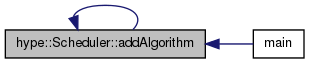bool hype::Scheduler::addObservation ( const SchedulingDecision & sched_dec, const double & measured_execution_time )
Parameters:
 sched_dec the scheduling decision, this observation belongs to measured_execution_time measured execution time, in nanoseconds!!!
Returns:
true on success and false in case an error occured
Examples:
online_learning_manual_time_measurement.cpp.

Definition at line 75 of file hype.cpp.

Here is the call graph for this function:Here is the caller graph for this function: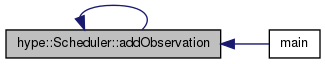hype::core::EstimatedTime hype::Scheduler::getEstimatedExecutionTime ( const OperatorSpecification & op_spec, const std::string & alg_name )

Definition at line 84 of file hype.cpp.

References getEstimatedExecutionTime(), and instance().

Referenced by getEstimatedExecutionTime(), and hype_getEstimatedExecutionTime().

Here is the call graph for this function:Here is the caller graph for this function:const SchedulingDecision hype::Scheduler::getOptimalAlgorithm ( const OperatorSpecification & op_spec, const DeviceConstraint & dev_constr )
Parameters:
 op_spec OperatorSpecification, contains all available information about the operator to execute dev_constr DeviceConstraint, restricting the available algorithms to a subset of the algorithm pool (e.g., allow only CPU algorithms)
Returns:
SchedulingDecision, which contains the suggested algortihm for the specified information
Examples:
online_learning.cpp, and online_learning_manual_time_measurement.cpp.

Definition at line 65 of file hype.cpp.

References getOptimalAlgorithm(), and instance().

Here is the call graph for this function:Here is the caller graph for this function: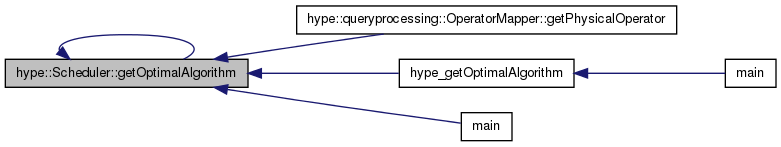Scheduler & hype::Scheduler::instance ( ) ` [static]`
Returns:
Reference to Scheduler instance.
Examples:
online_learning.cpp, and online_learning_manual_time_measurement.cpp.

Definition at line 21 of file hype.cpp.

Here is the caller graph for this function: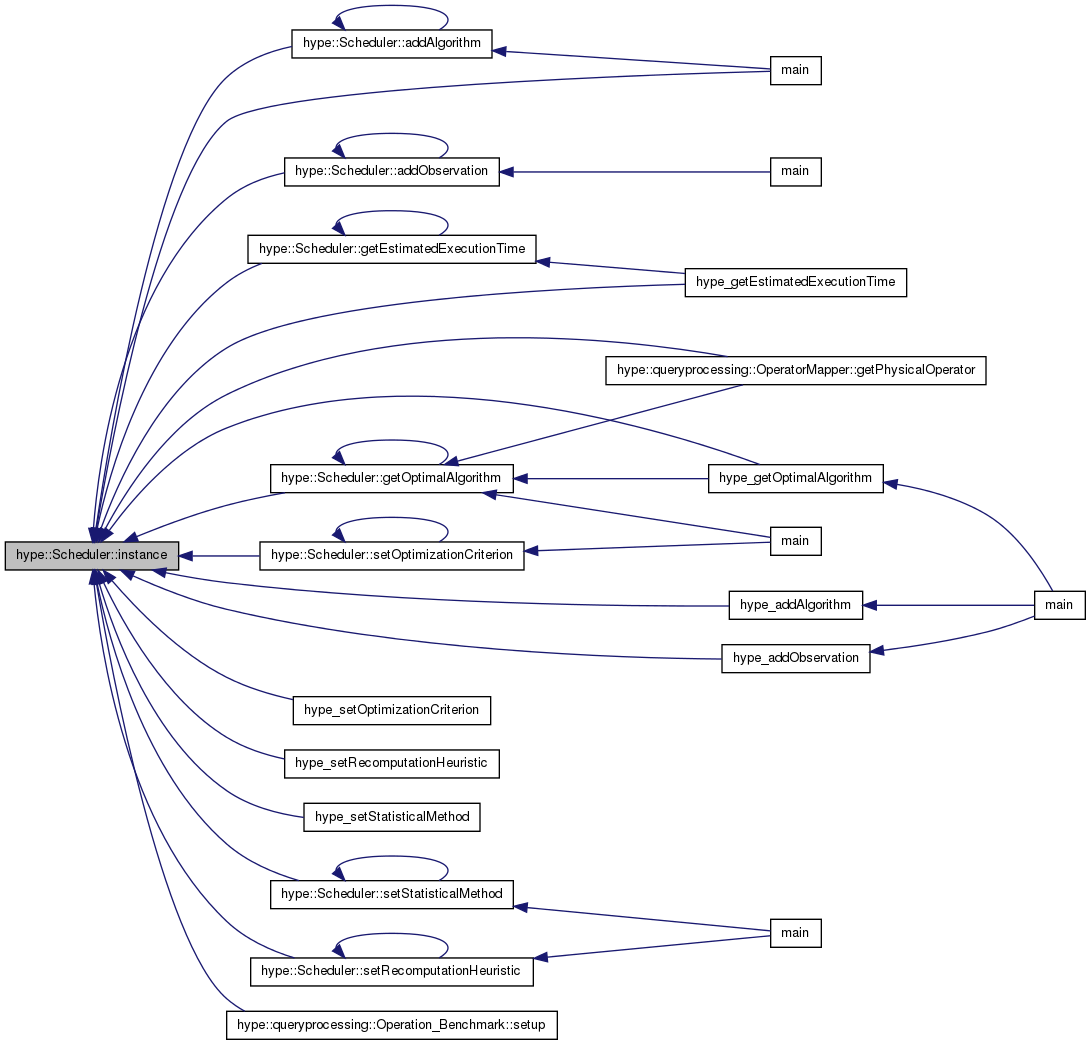Scheduler& hype::Scheduler::operator= ( const Scheduler & s ) ` [private]`
Parameters:
 reference to existing Scheduler object
 bool hype::Scheduler::setOptimizationCriterion ( const std::string & name_of_operation, const std::string & name_of_optimization_criterion )
Parameters:
 name_of_operation name of the Operation name_of_optimization_criterion Name of OptimizationCriterion
Returns:
returns true on success and false otherwise
Examples:
online_learning.cpp, and online_learning_manual_time_measurement.cpp.

Definition at line 41 of file hype.cpp.

References instance(), and setOptimizationCriterion().

Referenced by main(), and setOptimizationCriterion().

Here is the call graph for this function:Here is the caller graph for this function: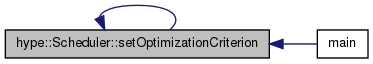bool hype::Scheduler::setRecomputationHeuristic ( const std::string & name_of_algorithm, const std::string & name_of_recomputation_strategy )
Parameters:
 name_of_algorithm Name of Algorithm name_of_recomputation_strategy assigns the RecomputationHeuristic name_of_recomputation_strategy to an existing Algorithm
Returns:
returns true on success and false otherwise

Definition at line 53 of file hype.cpp.

References instance(), and setRecomputationHeuristic().

Referenced by main(), and setRecomputationHeuristic().

Here is the call graph for this function:Here is the caller graph for this function:bool hype::Scheduler::setStatisticalMethod ( const std::string & name_of_algorithm, const std::string & name_of_statistical_method )
Parameters:
 name_of_algorithm Name of Algorithm name_of_statistical_method assigns the StatisticalMethod name_of_statistical_method to an existing Algorithm
Returns:
returns true on success and false otherwise

Definition at line 47 of file hype.cpp.

References instance(), and setStatisticalMethod().

Referenced by main(), and setStatisticalMethod().

Here is the call graph for this function:Here is the caller graph for this function: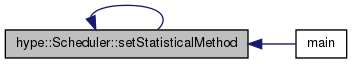The documentation for this class was generated from the following files: